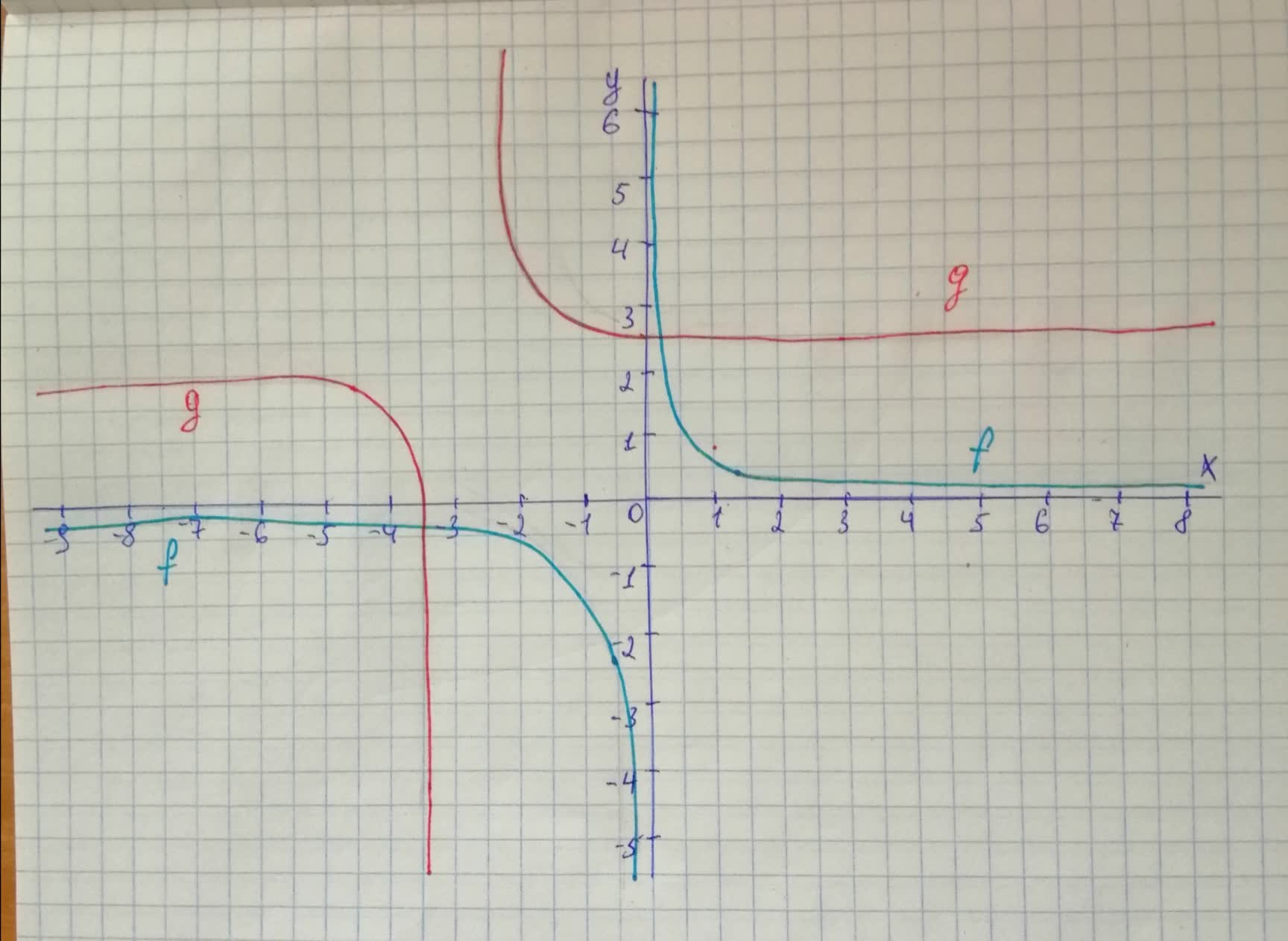# Use long division to rewrite the equation for g in the formtext{quotient}+frac{remainder}{divisor}Then use this form of the function's equation andvazelinahS 2020-12-21 Answered

Use long division to rewrite the equation for g in the form
$\text{quotient}+\frac{remainder}{divisor}$
Then use this form of the function's equation and transformations of
$f\left(x\right)=\frac{1}{x}$
to graph g.
$g\left(x\right)=\frac{2x+7}{x+3}$

You can still ask an expert for help

• Questions are typically answered in as fast as 30 minutes

Solve your problem for the price of one coffee

• Math expert for every subject
• Pay only if we can solve itkomunidadO

Step 1

The quotient is 2 and the remainder is
We can graph g by horizontal shifting f 3 units left then vertical shifting 2 units up
Step 2